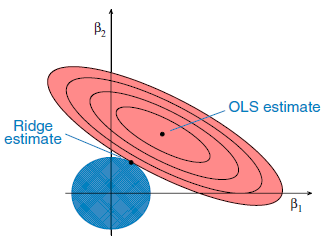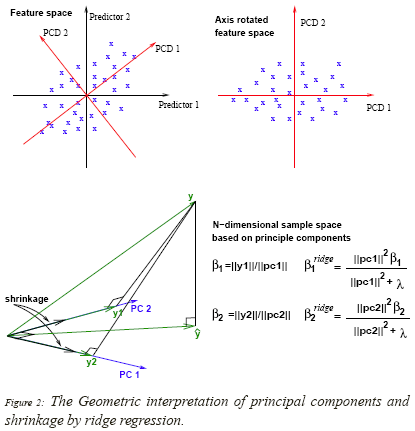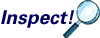# 5.1 - Ridge RegressionPrinter-friendly version

Motivation: too many predictors

• It is not unusual to see the number of input variables greatly exceed the number of observations, e.g. micro-array data analysis, environmental pollution studies.

• With many predictors, fitting the full model without penalization will result in large prediction intervals, and LS regression estimator may not uniquely exist.

Motivation: ill-conditioned X

• Because the LS estimates depend upon $(X'X)^{-1}$, we would have problems in computing $\beta_{LS}$ if $X'X$ were singular or nearly singular.

• In those cases, small changes to the elements of $X$ lead to large changes in $(X'X)^{-1}$.

• The least square estimator $\beta_{LS}$ may provide a good fit to the training data, but it will not fit sufficiently well to the test data.

Ridge Regression

One way out of this situation is to abandon the requirement of an unbiased estimator.

We assume only that X's and Y have been centered, so that we have no need for a constant term in the regression:

• X is a n by p matrix with centered columns,
• Y is a centered n-vector.

Hoerl and Kennard (1970) proposed that potential instability in the LS estimator

\begin{equation*}
\hat{\beta} = (X'X)^{-1} X' Y,
\end{equation*}

could be improved by adding a small constant value $\lambda$ to the diagonal entries of the matrix $X'X$ before taking its inverse.

The result is the ridge regression estimator

\begin{equation*}
\hat{\beta}_{ridge} = (X'X+\lambda I_p)^{-1} X' Y
\end{equation*}

Ridge regression places a particular form of constraint on the parameters ($\beta$'s): $\hat{\beta}_{ridge}$ is chosen to minimize the penalized sum of squares:

\begin{equation*}
\sum_{i=1}^n (y_i - \sum_{j=1}^p x_{ij}\beta_j)^2 + \lambda \sum_{j=1}^p \beta_j^2
\end{equation*}

which is equivalent to minimization of $\sum_{i=1}^n (y_i - \sum_{j=1}^p x_{ij}\beta_j)^2$ subject to, for some $c>0$, $\sum_{j=1}^p \beta_j^2 < c$, i.e. constraining the sum of the squared coefficients.

Therefore, ridge regression puts further constraints on the parameters, $\beta_j$'s, in the linear model. In this case, what we are doing is that instead of just minimizing the residual sum of squares we also have a penalty term on the $\beta$'s. This penalty term is $\lambda$ (a pre-chosen constant) times the squared norm of the $\beta$ vector. This means that if the $\beta_j$'s take on large values, the optimization function is penalized. We would prefer to take smaller $\beta_j$'s, or $\beta_j$'s that are close to zero to drive the penalty term small.

Geometric Interpretation of Ridge Regression:The ellipses correspond to the contours of residual sum of squares (RSS): the inner ellipse has smaller RSS, and RSS is minimized at ordinal least square (OLS) estimates.

For $p=2$, the constraint in ridge regression corresponds to a circle, $\sum_{j=1}^p \beta_j^2 < c$.

We are trying to minimize the ellipse size and circle simultanously in the ridge regression. The ridge estimate is given by the point at which the ellipse and the circle touch.

There is a trade-off between the penalty term and RSS. Maybe a large $\beta$ would give you a better residual sum of squares but then it will push the penalty term higher. This is why you might actually prefer smaller $\beta$'s with worse residual sum of squares. From an optimization perspective, the penalty term  is equivalent to a constraint on the $\beta$'s. The function is still the residual sum of squares but now you constrain the norm of the $\beta_j$ 's to be smaller than some constant c. There is a correspondence between $\lambda$ and c. The larger the $\lambda$ is, the more you prefer the $\beta_j$'s close to zero. In the extreme case when $\lambda = 0$, then you would simply be doing a normal linear regression. And the other extreme as $\lambda$ approaches infinity,  you set all the $\beta$'s to zero.

Properties of Ridge Estimator:

• $\hat{\beta}_{ls}$ is an unbiased estimator of $\beta$; $\hat{\beta}_{ridge}$ is a biased estimator of $\beta$.

For orthogonal covariates, $X'X=n I_p$, $\hat{\beta}_{ridge} = \frac{n}{n+\lambda} \hat{\beta}_{ls}$. Hence, in this case, the ridge estimator always produces shrinkage towards $0$. $\lambda$ controls the amount of shrinkage.

An important concept in shrinkage is the "effective'' degrees of freedom associated with a set of parameters. In a ridge regression setting:

1. If we choose $\lambda=0$, we have $p$ parameters (since there is no penalization).
2. If $\lambda$ is large, the parameters are heavily constrained and the degrees of freedom will effectively be lower, tending to $0$ as $\lambda\rightarrow \infty$.

The effective degrees of freedom associated with $\beta_1, \beta_2, \ldots, \beta_p$ is defined as
\begin{equation*}
df(\lambda) = tr(X(X'X+\lambda I_p)^{-1}X') = \sum_{j=1}^p \frac{d_j^2}{d_j^2+\lambda},
\end{equation*}
where $d_j$ are the singular values of $X$.  Notice that $\lambda = 0$, which corresponds to no shrinkage, gives $df(\lambda) = p$ (as long as $X'X$ is non-singular), as we would expect.

There is a 1:1 mapping between $\lambda$ and the degrees of freedom, so in practice one may simply pick the effective degrees of freedom that one would like associated with the fit, and solve for $\lambda$.

• As an alternative to a user-chosen $\lambda$, cross-validation is often used in choosing $\lambda$: we select $\lambda$ that yields the smallest cross-validation prediction error.
• The intercept $\beta_0$ has been left out of the penalty term because $Y$ has been centered. Penalization of the intercept would make the procedure depend on the origin chosen for $Y$.

• Since the ridge estimator is linear, it is straightforward to calculate the variance-covariance matrix $var(\hat{\beta}_{ridge}) = \sigma^2 (X'X+\lambda I_p)^{-1} X'X (X'X+\lambda I_p)^{-1}$.

A Bayesian Formulation

Consider the linear regression model with normal errors:

\begin{equation*}
Y_i = \sum_{j=1}^p X_{ij}\beta_j + \epsilon_i
\end{equation*}
$\epsilon_i$ is i.i.d. normal errors with mean 0 and known variance $\sigma^2$.

Since $\lambda$ is applied to the squared norm of the β vector, people often standardize all of the covariates to make them have a similar scale. Assume $\beta_j$ has the prior distribution $\beta_j \sim_{iid} N(0,\sigma^2/\lambda)$. A large value of $\lambda$ corresponds to a prior that is more tightly concentrated around zero, and hence leads to greater shrinkage towards zero.

The posterior is $\beta|Y \sim N(\hat{\beta}, \sigma^2 (X'X+\lambda I_p)^{-1} X'X (X'X+\lambda I_p)^{-1})$, where $\hat{\beta} = \hat{\beta}_{ridge} = (X'X+\lambda I_p)^{-1} X' Y$, confirming that the posterior mean (and mode) of the Bayesian linear model corresponds to the ridge regression estimator.

Whereas the least squares solutions $\hat{\beta}_{ls} = (X'X)^{-1} X' Y$ are unbiased if model is correctly specified, ridge solutions are biased, $E(\hat{\beta}_{ridge}) \neq \beta$. However, at the cost of bias, ridge regression reduces the variance, and thus might reduce the mean squared error (MSE).

\begin{equation*}
MSE = Bias^2 + Variance
\end{equation*}

More Geometric Interpretations (optional)

• Inputs are centered first;
• Consider the fitted response
• \begin {align} \hat{y} &=\textbf{X}\hat{\beta}^{ridge}\\ & = \textbf{X}(\textbf{X}^{T}\textbf{X} + \lambda\textbf{I})^{-1}\textbf{X}^{T}\textbf{y}\\ & = \textbf{U}\textbf{D}(\textbf{D}^2 +\lambda\textbf{I})^{-1}\textbf{D}\textbf{U}^{T}\textbf{y}\\ & = \sum_{j=1}^{p}\textbf{u}_j \frac{d_{j}^{2}}{d_{j}^{2}+\lambda}\textbf{u}_{j}^{T}\textbf{y}\\ \end {align}

where $\textbf{u}_j$ are the normalized principal components of X.

• Ridge regression shrinks the coordinates with respect to the orthonormal basis formed by the principal components.
• Coordinates with respect to principal components with smaller variance are shrunk more.
• Instead of using X = (X1, X2, ... , Xp) as predicting variables, use the new input matrix $\tilde{X}$ = UD
• Then for the new inputs:
• $\hat{\beta}_{j}^{ridge}=\frac{d_{j}^2}{d_{j}^{2}+\lambda}\textbf{u}_{j}^{T}\textbf{y}$

$Var(\hat{\beta}_{j})=\frac{\sigma^2}{d_{j}^{2}}$

where $\sigma^2$ is the variance of the error term $\epsilon$ in the linear model.

• The shrinkage factor given by ridge regression is:

$\frac{d_{j}^{2}}{d_{j}^{2}+\lambda}$

We saw this in the previous formula. The larger λ is, the more the projection is shrunk in the direction of $u_j$. Coordinates with respect to the principal components with a smaller variance are shrunk more.

Let's take a look at this geometrically.This interpretation will become convenient when we compare it to principal components regression where instead of doing shrinkage, we either shrink the direction closer to zero or we don't shrink at all. We will see this in the "Dimension Reduction Methods" lesson.